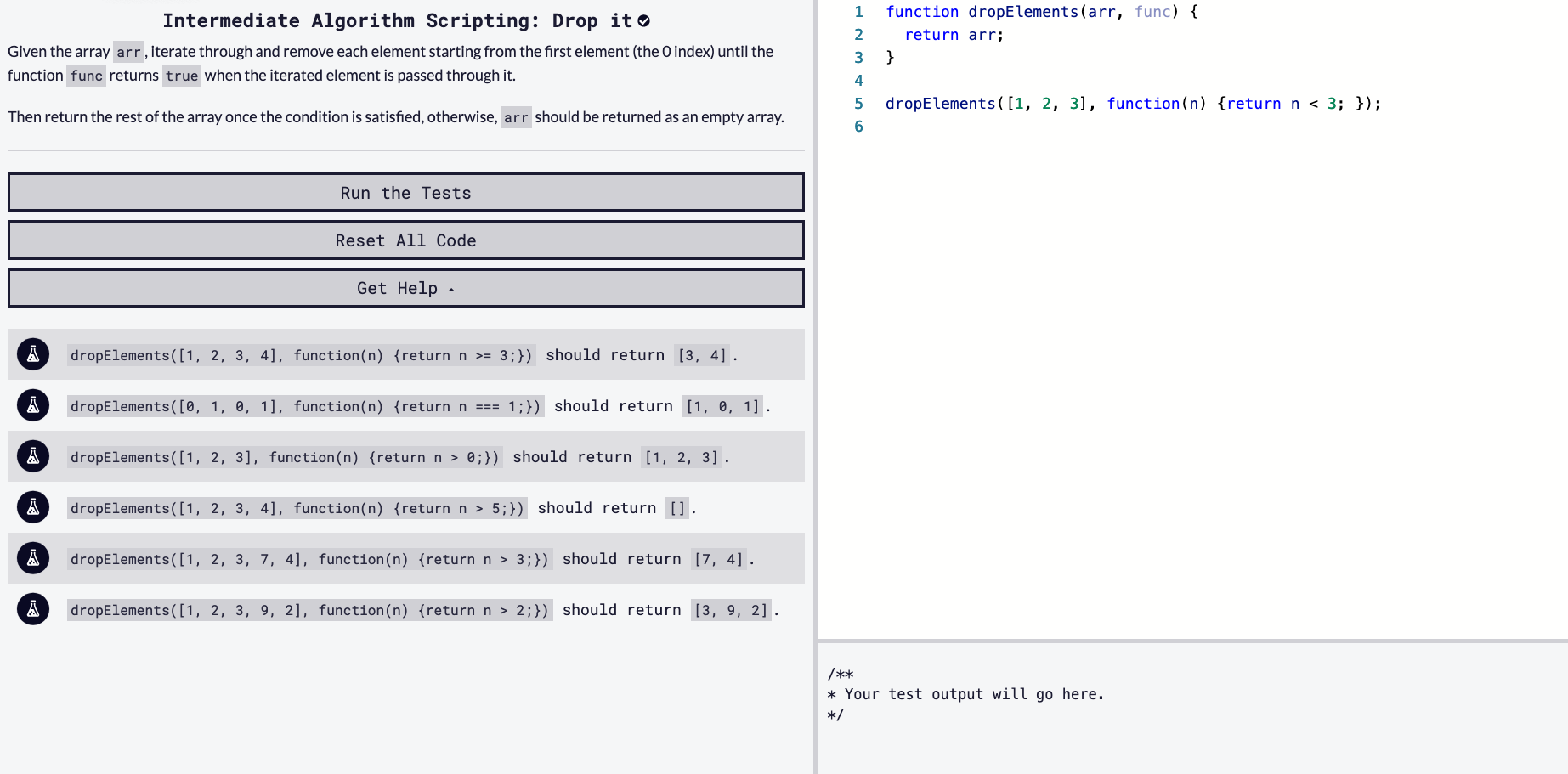## DEV Community

Viren B

Posted on • Originally published at virenb.cc

# Solving "Drop it" / freeCodeCamp Algorithm ChallengesLet's solve freeCodeCamp's intermediate algorithm scripting challenge, 'Drop it'.

### Starter Code

``````function dropElements(arr, func) {
return arr;
}

dropElements([1, 2, 3], function(n) {return n < 3; });
``````

### Instructions

Given the array `arr`, iterate through and remove each element starting from the first element (the 0 index) until the function `func` returns `true` when the iterated element is passed through it.

Then return the rest of the array once the condition is satisfied, otherwise, `arr` should be returned as an empty array.

### Test Cases

• `dropElements([1, 2, 3, 4], function(n) {return n >= 3;})` should return `[3, 4]`.
• `dropElements([0, 1, 0, 1], function(n) {return n === 1;})` should return `[1, 0, 1]`.
• `dropElements([1, 2, 3], function(n) {return n > 0;})` should return `[1, 2, 3]`.
• `dropElements([1, 2, 3, 4], function(n) {return n > 5;})` should return `[]`.
• `dropElements([1, 2, 3, 7, 4], function(n) {return n > 3;})` should return `[7, 4]`.
• `dropElements([1, 2, 3, 9, 2], function(n) {return n > 2;})` should return `[3, 9, 2]`.

# Our Approach

After reading the instructions, starter code, and test cases more than once, this is what we're working with:

• Our function takes in two arguments, an array (`arr`), and a function (`func`). `arr` consists of numbers.

• We must return an array.

• We want to alter `arr` based on the constraints of `func`, then return `arr`.

I had to read the instructions a few times to get a better idea of what the challenge was actually asking for. We want to work with `arr`, and remove each index until we come across a truthy index. So let's explore one of the test cases first off:

``````dropElements([1, 2, 3, 4], function(n) {return n >= 3;}) // should return [3, 4]
``````

So, based on the arguments, we want to evaluate `arr` with `func` and keep the values that are greater than or equal to 3. Seems some what straight forward. Let's look at the last test case provided though:

``````dropElements([1, 2, 3, 9, 2], function(n) {return n > 2;}) // should return [3, 9, 2].
``````

We want values greater than two so why is  being returned? Have a read at the below instruction:

Then return the rest of the array once the condition is satisfied, otherwise, `arr` should be returned as an empty array.

So once we hit something truthy, we'll return all the following elements. This is a more clear example:

``````dropElements([8, 1, 2, 3], function(n) {return n > 3; });

// On arr, we have 8. 8 is greater than 3. Even though 1 and 2 are not greater than 3, arr has already met the constraint from the function so we return the rest of the elements.
``````

Alright, hopefully the instructions are making a little more sense now. Let's get into the code.

The first thing I will do is set a variable equal to `arr`'s length so we know how many times to loop.

``````const arrLength = arr.length;
``````

I think it is time for the `for` loop.

``````for (let i = 0; i < arrLength; i++) {}

// We'll start i at 0 since array's index begins at 0
``````

The next thing we'll need is an `if` statement to see if each index meets the constraint provided in the second argument, `func`. `func` takes in one argument, `n`. We can use `arr` as `func`'s argument each loop to see if it will meet the constraint.

``````for (let i = 0; i < arrLength; i++) {
if (!func(arr)) {
// more code coming
}
}
``````

Above, I'm using `arr` instead of `arr[i]` because of the code we will be putting inside the `if` statement. If `func(arr)` is false, we will remove it, right? Enter the array method, `shift()`. `pop()` or `push()` are common but here we want to remove elements from the beginning of the array and that is what `shift()` does for us.

``````for (let i = 0; i < arrLength; i++) {
if (!func(arr)) {
arr.shift();
}
else {
return arr;
}
}
``````

So let's try this step by step with one of our test cases:

``````dropElements([1, 2, 3, 4], function(n) {return n >= 3;});

const arrLength = arr.length // 4
for (let i = 0; i < arrLength; i++) {
if (!func(arr)) {
arr.shift();
}
else {
return arr;
}
}

// Loop 1: arr = 1; is not greater or equal to 3. shift() run
// Loop 2: [2,3,4]; arr = 2; not greater or equal to 3. shift() run
// Loop 3: [3,4]; arr = 3; 3 is greater than or equal. return arr
``````

As always, return `arr` and that is it!

# Our Solution

``````function dropElements(arr, func) {
const arrLength = arr.length;
for (let i = 0; i < arrLength; i++) {
if (!func(arr)) {
arr.shift();
}
else {
return arr;
}
}
return arr;
}
``````

'Drop it' Challenge on fCC

freeCodeCamp

Donate to FCC!

Solution on my GitHub